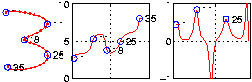# Digit Recognition in Curvature Space

Manolis Kamvysselis

# Introduction

## Abstract

This paper presents our experience with an curvature space for online character recognition. The (x,y) coordinate data along the character curve is transformed to local curvature data. The peaks of this curvature represent the sharp turns made by the pen in constructing the character. The height and timestamp of these peaks are used as features. The number of peaks for a single character rarely exceeds five or six. Thus, we have transformed the 256 dimensional data of off-line recognition on a 16x16 grid, to less than 10 dimensional data.

This aggressive reduction in dimensionality is certainly lossy. However this paper makes the claim that the curvature peaks preserve the information most important to character structure. To demonstrate the claim, we construct a classifier for handwritten digits that bases its decisions solely on the few peaks in curvature space.

The results are promising: 200 digits of each character were correctly classified based on a HMM mixture model constructed from 300 examples of each digit. Unsupervised learning techniques are also envisioned. The character data plotted in the lower dimensional space suggests distinct clusters emerge, that could separate and group trained symbols, as well as recognize new symbols as categories.

## Algorithm Overview

The first step of the algorithm is a compression problem, to reduce the dimensionality of the data, while maintaining a meaningful set of features. The (x,y) character data set is first transformed to (dx,dy) increments along the path of the pen. These increments are transformed into (angle, distance) increments, and finally the derivative of the angles data is used for the representation. Our measure of the curvature at a point in the character curve will be the value of the angle derivative at that point. A very important denoising step is applied to this derivative data, to filter out unimportant variations and bring out the meaningful variations. The peaks in this denoised derivative show the points of highest curvature. These points correspond to the moments when the pen was changing direction, in other words, the transitions between consecutive sub-strokes within the same character.

These points of highest curvature are used as features. The set of (derivative, index) pairs for each peak are what we base our recognition upon. We train an HMM network to cluster the data from each character. The representation for a character becomes then a mixture of Gaussian centered on the clusters of points in the (derivative, index) space. A new character is classified by comparing the likelihoods obtained by each of the models when explaining the peaks of this new character.

## Organization of the paper

The next part of the paper gives an overview of data preprocessing steps. The third part outlines the transformation to curvature space. The fourth part of the paper explains the noise reduction mechanism and its performance. Section 5 details the feature selection step. Section 6 goes over the training of the HMMs on the data. Section 7 evaluates the performance of the algorithm. Section 8 concludes with applications of this work and possible extensions. All the figures have been provided in appendix, so that they can be made as large as possible without interference with the text. The legends of the figures will be given within the text.

# Working with online data

The online character data was kindly provided by Nick Matsakis (matsakis@ai.mit.edu). It contains 500 examples of each of the 10 digits obtained on a Cross handwriting recognition pad. Nick was preprocessing the data, and we kept his preprocessing code. He resamples the data uniformly, along the curve, to obtain the same number of points for all data samples. This processing step will not be described in this paper.

After uniform resampling, each character in the data set has 36 points, in order, in (x,y) coordinate form. All characters have the same length, and they are centered at zero. Their orientation is not normalized however, and the same character could be rotated in different ways.

The i coordinate is an index from 1 to 36, implicitly provided by the ordering of the points. The problem of online data recognition seems to at least as easy as off-line recognition, since one can always simply drop the i indices, and fall back onto an off-line recognition problem, where the order of points is not known.

## Relation with Offline Data

The problem is that even though the keystrokes of two people when writing a 4 can be different, their written outputs are usually similar. This is because we humans have tailored our writing to match the expectations of an off-line recognizer, namely other humans. We are taught to write so that the pixel output looks like the desired character, regardless of what series of steps (strokes) we take to achieve it. People often optimize for speed and ease of writing consecutive characters, so the order ends up being often the same across different writers. This work didn't have to cope with this problem, since Nick Matsakis has preprocessed the data to reverse each non-regular stroke. Some irregularities remain, of course, and they will not be solved by simple preprocessing steps. We accept them though as different representations of the same character.

This section compares the two problem domains, and shows how the dimensionality of online data is intrinsically lower. The next section will describe how we further reduce this dimensionality in angle derivative space.

Online data for character recognition is intrinsically easier to deal with than pixel data. We are a step closer to the production of characters, since the information about individual stokes has not been lost on a static paper (or pixel-map). We have all the information about the curves that the pen traverses in space, in order to write the character down, and we can use the structure of this curve to our advantage.

In fact, since characters can be recognized independent of their scale, we can guess that spacing of the different points is not what is important in the recognition process. The only time when scale enters in the recognition process is when multiple characters are placed next to each other and a capital O for example, is distinguished from a lower case o. In fact, if we sample at equidistant points along the curve

## Online data is lower-dimensional

We will take advantage of the fact that online data is available to reduce the dimensionality of our problem. Compare a pixel-based character recognition dimensionality in a 16 by 16 grid. Each pixel can be on or off, a total of 256 dimensions. However, a much smaller number of actual data points was used in creating the character. Principal components analysis on the 256 dimensional data reveals that with only 30 dimensions the data can be represented accurately enough to yield less than 10% classification error. In fact, our online data has an (x,y) coordinate for each of the 36 samples, hence 72 dimensions. If we disregard lengths, and only keep angle data, we have come down to 36 dimensions. This seemed to be the minimum given by PCA. Going further seems pointless, since classification seemed to deteriorate greatly with anywhere less than 10 dimensional data.

# Character Transformation to Angle Derivative Space

## Getting familiar with angle derivative space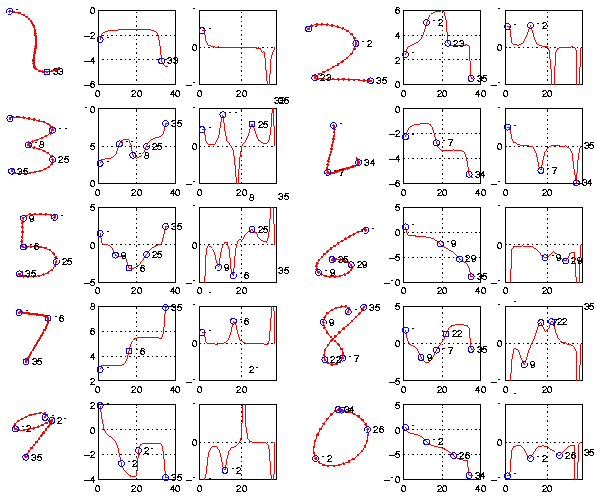We encourage the reader to take a moment now to look at the figures in the appendix. Three types of plots are presented in figure 1. The first column (and the fourth) show the means of the 500 samples of each character in our data set. The characters were averaged per index. That is the coordinates of the third point of digit 5, for example, are the average of all the coordinates of point 3 of every sample of digit 5.

The first thing to notice is how smooth these characters look. If characters looked like that out of the character capture system, then our recognition task would be much easier. We will show how denoising can restore smoothness to original curves in the next section.

The second thing that strikes is that the first character doesn't look anything like a one, and the fourth seems to be missing something. The explanation for digit 4 is simply that nick has software that handles single stores, so we only get the first stroke of a two-stroke 4, missing the vertical bar that was coming next. As for digit 1, we postulate that this curved shape comes from the fact that it is an average over different orientations of 1.

Now, one can go more into detail in understanding the angle derivative representation intuitively. Take the example of character 3. After an initial discontinuity that we ignore as noise when the pen of the user is put on the table, the derivative goes up, then it decreases sharply, then it goes up again, and finishes with a discontinuity that we ignore again as noise before the pen of the user leaves the table. The increase and then decrease in derivative values corresponds to a right turn along the path of the stroke. The narrow and highly negative value corresponds to a sharp left turn. Then the right turn is repeated for the second loop of the three. The peaks in the derivative and their corresponding turns on the character are circled in purple on the figure. I would recommend going over the different characters and understanding those peaks to get familiar with the representation before proceeding further in the paper.

Figure 2 shows the same peaks that were highlighted by hand in figure 1, picked out automatically by the algorithm in the angle derivative space, and reported onto the angle space, and the character curve. The derivative axes are enforced to go from -1 to 1, to make comparison between derivatives across character easier. This chops off some derivatives that were higher than 1. We allowed those curves to extend pas the axes on figure 2b.

## Motivation for an angle derivative space

Now that this angle space is more familiar, let's first see the motivation behind it as a representation for digits. First of all, one needs to point out that the reason why pixel-based classification (and even PCA dimensionality reduction) worked so well with little effort is that the data had already been normalized. First of all, the data was centered on zero, since pixel-based approaches are location specific. Then the data was scaled so that it has total length 1. Finally Nick went through great effort to get the character representation invariant to rotation. He applies a linear transformation (a combination of rotation, scaling, shear) to every character in its curve form in order to align it optimally with the rest of the data, before it was transformed onto pixels. The hard part was to get atransformation that is not character specific, and hence can be used for classification (as opposed to a transformation specific to threes, for example). What makes the (x,y) coordinate space unfit for recognition is that uniform global modifications, such as rotation, transform the space in a non-uniform way. Different coordinates will change in different ways, which makes recogntion hard without a sometimes involved normalization step that should get rid of irrelevant noise while preserving features important to recognition.

A curvature approach is inherently translation invariant, scaling invariant, and rotation invariant. Under any of these transformations of the input set, the representation will be the same. The angles taken in this representation are not global angles of every point with respect to say the center of the character, but instead local angles on the coordinate derivatives from one point to the next. When a character is rotated, the angles all shift together, since they are based upon increments to the angle of the first segment of the character. Hence, global transformations of the character do not transform these local angles. We argue therefore that an angle derivative space is best fit to a character recognition problem, being invariant to changes in the input that are irrelevant to recognition, and making recognition relevant features stand out, such as the three curves in the digit 3, along with their sharpness and direction.

## Resolving angle ambiguities

The main problem of an angle space are the ambiguities due to the periodicity of the data. In a space constrained between 0 and, when an angle varies smoothly from 6.2 to 6.3, the angle seems to jump fromdown to nearly 0 causing jumps in the derivative. To get around this problem and obtain smooth data plots, we allow the angles to cross the boundaries, but force them to preserve smoothness in transitions. That is when we have to choose which equivalent representation to give to the next angle down the line, we will choose the one that is most similar to the current one. In other words we add or subtract a multiple ofto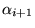, to enforce(1)

Angle derivatives have a range of, whereas angles have a range of. Intuitively, think of moving around the unit circle. If you ever have to take a step great thanin one direction to reach a particular point on the unit circle, you can simply move in the opposite direction, and the distance to travel will be now less than, since the two ways of getting there must sum up to.

When this smoothness constraint is applied to the angle derivatives, the corresponding angle plots can span ranges greater than.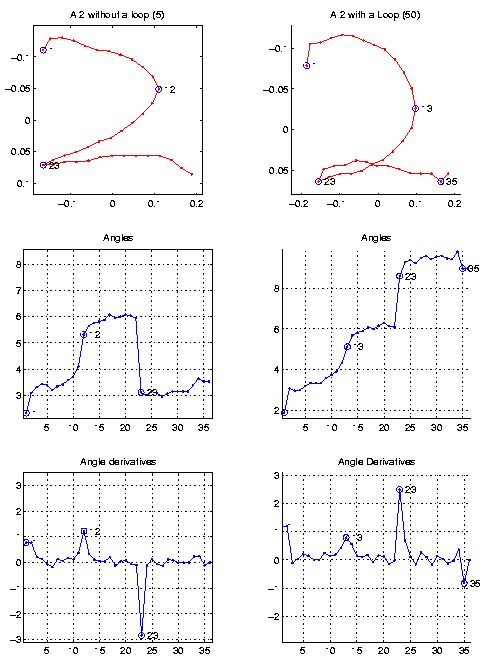An example of such a plot can be found in figure 4. When digit 2 is drawn with a loop, the curvature along the curve never changes sign. In logo terms, this 2 could be drawn by a turtle that keeps turning right. After the turtle turns 360 degrees, it is facing back in the original direction, but continues to turn, which yields a span greater than.

We seem to have cured therefore what seems to be a great problem with angle plots, that two curves could be very similar, and yet their angle plots could look very different because of the periodicity of angle space. Now up to a constant shift along the y axis, two curves of similar characters will look the same.

## The loop ambiguity

Figure 4 illustrates another ambiguity that we need to account for. In a sharp corner, such as the bottom left corner of a 2, or the middle curve of a 3, writers will draw a loop instead of a sharp corner. These loops are sometimes important to recognition. For example, imagine keeping only the bottom part of the twos in figure 4 (after indices 12 and 13). The bottom of the left digit can be interpreted as a less than character (<), whereas the bottom of the right digit can be interpreted as the greek letter alpha (). This shows that our eyes are not indifferent to a loop when we see one, and the two kinds of twos are different characters, both representations both as the number 2. In fact when i came to the US, I personally had a hard time recognizing American 2s with overemphasized lower loops. Hence we could simply consider the two characters to be different, and perhaps an unsupervised clustering algorithm would instruct us so. When we get a little further in the paper, we will show how the loops appear in our learning system and how we deal with them.

This loop ambiguity explains an artifact we see in figure 3. Figure 3 shows the average characters, this time averaged over angle derivative space. We see that orientation is sometimes not preserved (in the case of 7, 9, and 6), but that otherwise, the characters look very natural (in the case of 3, 5, 8, 0, and even the tilted 7, 9 and 6). The major concern is the averaged 2 which is missing its bottom loop. The loop has degenerated to an almost straight line (some indication of an angle shows at the position indicated by the arrow, but there is clearly something wrong). What goes wrong is the fact that we are averaging angles in a euclidian space, as opposed to a periodic space more natural to angles. Hence, the two types of twos shown in figure 4, having derivatives similar in magnitude but opposite in sign, will cancel each other and leave the averaged angle derivatives of the 2 almost flat at that point in the curve (the second turn of 2 went from being the dominant feature in both cases of figure 4, into being much smaller than the smaller peak in the original character).

In fact, this loop ambiguity is very easily interpreted in the derivative angle space. The values for the derivatives at point 23 seem very different when laid out on the plane, but they are in fact close in angle derivative space. One should think of the angle graphs as wrapping around themselves along the y dimension, as if they were the unwrapped side of a cylinder laid out on the paper. Since our smoothness constraint (equation 1) ensures that derivatives will be constrained within a range of, the space wraps atand, and very negative derivative values are close to very positive derivatives. The error function in our learning algorithm should be able to handle this property of periodic variables. Our cost function should incorporate some notion of periodicity of the form(2)

which would yield an error function of(3)

along the angle dimension (to be then combined with errors from other dimensions).

## Working with Periodic Cost functions

We are not the first ones facing the problem of error estimation in an angle space. Solutions have been proposed for dealing with periodic cost functions. Bishop and Legleye (1995) propose an approach based on a mixture of kernel functions, that are themselves periodic. They replace a Gaussian kernel based on the x,y coordinates of speed vx and vy(4)

with a new distribution of the form(5)

known as the circular normal or von Mises distribution, where I0 is the zeroth-order modified Bessel function of the first kind.

An alternative is suggested in an exercise in Bishop's book (exercise 6.8 p.249). A density function is constructed for the periodic variableby replicating the rangean infinite number of times to construct a density in therange.(6)

This density allows comparison between similar valueswithout extra effort once the new distribution has been constructed. To make this approach practical the summation can be restricted to finite values of L. This will give a good approximation for Gaussian models with exponentially decaying tails.

In our case however, the difference between small negative curvature and small positive curvature matters. Negative curvatures correspond to left turns and positive curvatures to right turns in the character path. On the contrary nearpositive curvatures are very similar to nearnegative curvatures, because of the loop ambiguity. Hence, even though our representation angle derivatives goes from -pi to pi, we will do comparisons into the space from 0 to 2pi, with negative angles mapped accrordingly. Hence our error function becomes:(7)

where(8)

Moreover, we only consider angles greater than a threshold (.4)since small angles could simply be due to noise in the input. Hence, we will not introduce a lot of near-zero angle derivatives which would occupy the two ends of our new space.

## Enriching the representation

We would like our character transformation to be reversible. That is, we would like to be able to recover the character from the angle derivative representation. The reasons for this are multiple. First, we would like to check that our representation is correct. Looking at the quality of the reconstruction we can obtain, we can judge of the quality of the representation, and have a measure of information loss.

Secondly, we want to process the signal obtained in the angle derivative space, and judge what these changes correspond to in the character domain. Signal processing steps could be compression, noise reduction, smoothing, or resampling.

The derive operation is lossy, losing a constant at every order. Hence we need to enrich the representation with an angle constant, representing the orientation of the original character. Moreover, the position derivative from which we calculate the angles loses the absolute (x,y) position of the original character, and lastly moving to angle space loses the lengths.

To enable reconstruction, we add four constants to our representation.

• The (x,y) coordinates of the centroid of the original character.
• The mean segment length of the original character. If the sampling was uniform, this should be enough to recover the original character. Often it will not be an exact reconstruction, but the difference is very small and often imperceptible in our experiments.
• The orientation of the original character, explained below.

The orientation is encoded as the principle angle of the original character. We could have included any particular angle, to enable moving back from the derivative angle space to the angle space. However, since after signal processing particular angle derivatives can change, relying on a single value for the reconstruction would not have generated a stable representation invariant to noise. Hence we decided to use a value for a representative angle of the original character. This angle is given by the largest eigenvector of the original character. We perform a principle component analysis of the (x,y) coordinates in the original character, and find the direction and angle of maximal variation.(9)

where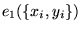is the eigenvector corresponding to the largest eigenvalue of the covariance matrix of all coordinate pairs in the original character.

Our reconstruction will be constrained to preserve the angle of that principle direction. To enforce that constraint, after reconstruction, we calculate the principle angle of the reconstructed point set, and adjust all the angles by the difference in the angles of the two principle angles of the original character and of the reconstructed character. The angle update is(10)

where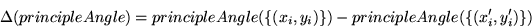(11)

where (xi',yi') are the reconstructed points.

The results of adding these four constants to our representation worked effectively in making the transformation reversible and the reconstructed characters almost identical to the original ones.

# Noise Reduction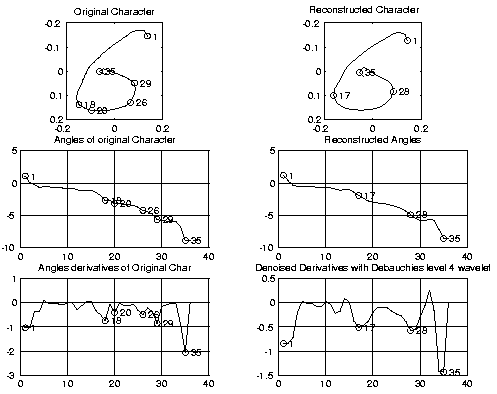When the entire character set is averaged, the curves obtained for the character, as well as their angles are very smooth. This creates very clean derivatives, with distinct peaks, which let us guess that recognition can be done in the derivative space. However, when a single curve is considered, the signal is often too noisy to find the distinct peaks. We had to find a solution that would filter out the high-frequency variations due to the shaking of the user's hand. To illustrate, we would like to go from a highly noisy signal for a 6 such as the one on the left of figure 5, to a clean signal like the one on the right of figure 5, that resembles much more closely the signal for the average 6 character, shown in figure 1.

## Fourier methods for denoising

A low pass fourier filter for noise reduction did not work, since the highest derivatives are necessary for encoding features in our curvature space. The fourier analysis did not seem to distinguish between the useful high frequencies due to features in the character transcribed from those high variations created when entering characters to the computer.

An experiment to try would be to reconstruct the curvature signal from a mixture of low frequency and high frequency components. We did not have time to perform this experiment.

## Brute force compression for denoising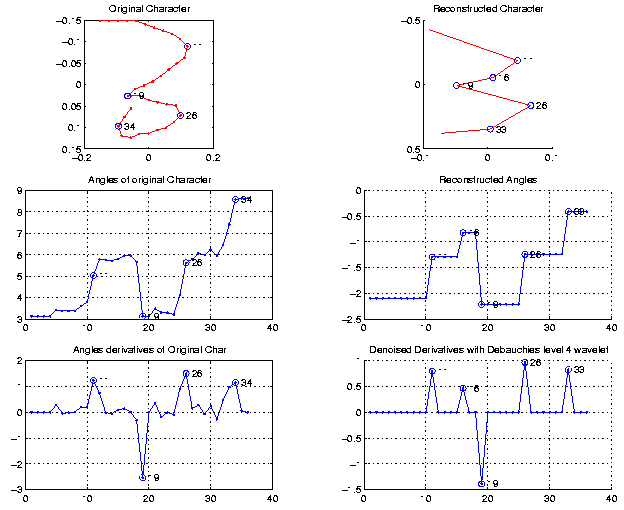Another form of denoising would be to completely eliminate the derivatives below a certain threshold. Figure 6 shows an example of such a aggressive compression trasnformation. The derivatives below 0.4 were simply set to zero. This preserves the most important variations of the curve, at the peaks of curvature, but the smoothness of the curve is lost. There is a low-frequency stability that should be preserved in the character, and it was destroyed in this case. The obtained reconstruction is very coarse (top right of figure 6).

The extra peak at 16 appears from a pre-processing to the aggressive compression step. This preprocessing is in fact the wavelet based denoising filter that we describe next. This step not only added a peak to our reconstruction of the 3, but it also lowered the peaks of the angle derivatives, by smoothing out the signal. The next section provides more details on the denoising.

## Wavelets reduce noise and bring out features in the curve

The hardest part in character recognition is noise reduction. If characters didn't have the meaningless variation due to noise, then we would simply use a nearest neighbor classifier and match the closest labelled curve every time a new perfect character was presented.

We argued that the angle space is useful for recognition but our argument won't hold unless we show that noise reduction is easy in that domain. In fact, using wavelets, the noise reduction step has very nice results, yielding a smoothing of the curves in curvature space, and thus very cleanly marked features with few irregularities.

We use a 4th order daubechies wavelet basis for noise reduction. The properties that make this wavelet basis a good choice is the fact first of all that it is an orthogonal basis with a compact support. We used soft thresholding based on a principle of Stein's unbiased risk threshold selection rule. We experimented with other wavelet bases and error functions, but the best performance was obtained with the combination described.

To judge the performance well the noise reduction works, we compare the curves obtained by noise reducing individual characters with the smooth curves obtained on the average characters. If the curves exhibit the same features, the recognition step will be possible.

In our case, the features are the peaks in the angle derivatives. These peaks occur when the angle derivative is maximal, that is when at the local minima of curvature along the character curve. We circle the peaks and number them with the index of the point at the peak in the derivatives, the angles, and the character (see figure 5).

The original signal had many local minima of curvature that was making it difficult for features to stand out. The denoised version of the signal has clearly marked peaks, that occur precisely where the average character had its peaks (compare with character 6 in figures 1 and 2). The method seems to be robust and the results are promising. Classification performances without this denoising step and with this denoising step are exhibited below.

 without denoising after denoising ones 87.96% 94.0541% twos 70.24% 87.8679% threes 83.68% 97.8378% fours 39.4% 53.5736% fives 97.64% 80.1802% sixes 83.8% 73.9339% sevens 3.56% 2.8228% eights 35.2% 71.4114% nines 70.12% 86.1261% zeros 70.48% 82.9429%

# Feature Selection

Once this representation is fixed, and we have successfully reduced the noise in the data, we have to extract features for recognition. Up until now, the transformation was lossless. An exact version of the character could be retrieved from the curvature angle derivative representation, given the appropriate lengths. The noise reduction step is not applied in the construction of the representation, but as a prerequisite to extracting the features, in order to make the features extracted independent of the input noise. Moreover, we showed that a good reconstruction of the original character can be obtained even after denoising. Hence up until this step all (or at least most) of the information in our signal has been preserved.

We define features as partial views of the data. Selecting features according to some criterion amounts projecting it onto a particular view, which usually greatly simplifies the data. A good property of a feature is comparability. That is feature extraction should enable comparison between characters by simple comparisons on the features.

From the curvature representation, we can extract the peaks after the noise-reduction step. For each peak i, we obtain an (indexi, heighti) pair. Each (index,height) pair becomes a feature.

Notice that the number of features is variable according to the number of peaks that the noise-reduction/peak-finding combination will extract. We will construct a model for each character. Then when a new sample is to be identified, we will see how each model explains the set of features in the sample. The model that explains the combination of features best will be chosen as the most likely category that the sample belongs to.

In figure 7 we plot the features extracted for each of the characters.

Notice that the heights of the peaks now go from 0 to, since we have applied the transformation of equation 8 to our data, so that high positive peaks and high negative peaks lie close together, while making sure that small negative values and small positive values are separated (see discussion in section 3.5).

Moreover, we have crossed out the peaks occuring within in first 5 and the last 5 points of each sample. This refers again to our discussion in section 3.1. The high derivatives in these points are due to the noise generated when the pen is laid on the table and when the pen is lifted from the table.

Also, we have circled in red the clusters of data that we think a clustering algorithm should pick out. This will help a quicker grasp of the information on this graph. Figure 7a has the regions of interest in an orange square, and figure 7b shows the same figure unedited. We invite the reader to compare the indices and heights of these peaks with those circled in purple in figure 1. For example, we can see all three peaks of character 3, stand out again. The first peak around 10 has a variation of 3 indices in where it occurs, anywhere from 8 to 13. In figure 2, we see this peak occuring at index 11 on the mean character. Hence, this graph provides us not only with the means of the features, but also an estimate of the variation of each feature.

Finally, noteworthy are also the peaks circled in orange. They show that some peaks of 2 and 3 have greater variation in the height of the peaks. This is the artifact of the loop ambiguity that we covered in section 3.4. The height of the peak depends (1) on the presence or absence of a loop, and (2) on the smoothness of this loop. For a 2, the top red circle shows presence of a loop with low curvature, apparently the most likely. The bottom red circle shows no loop and small curvature. Finally the two circles in orange are the high curvature samples, on the top with a loop, and the bottom without. Similar artifacts appear for the crossing of digit 9, and the midpoint of digit 5. These spreads occur for characters that require a sharp angle from the writer, who could stop the pen and hesitate on the curve to follow after that high curvature point. When charaters are written rapidly and repetitively, the curve can be smoothed out, include a loop, or a sharp corner. We encourage the reader to analyse similary the circles for digits 3, 5, and 9.

# Supervised Learning

## Training a mixture for each character

We train a Hidden Markov Model for each of the characters. Each state is made of two components, the index and the height of the peak. Priors for each state are calculated, and with transition probabilities between states. For each character, we train 5 hmm models for different numbers of states (from 2 to 6). Each state can be represented as a gaussian with a height corresponding to the likelihood of the particular center.

We use an EM clustering algorithm to find the centers of the gaussians in order to best explain the data. I didn't use the EM clustering code of problem set 2, but instead I downloaded a matlab implementation of HMM code. (Zoubin Ghahramani http://www.gatsby.ucl.ac.uk/zoubin/software.html).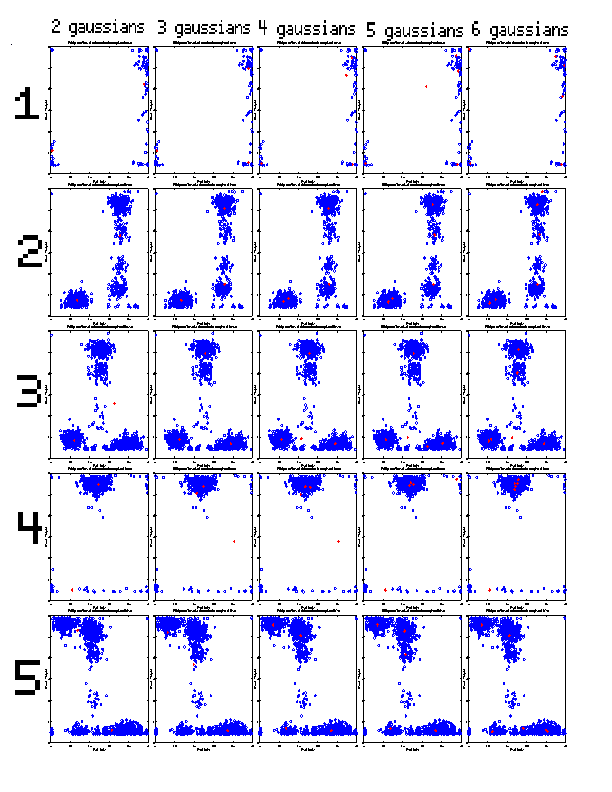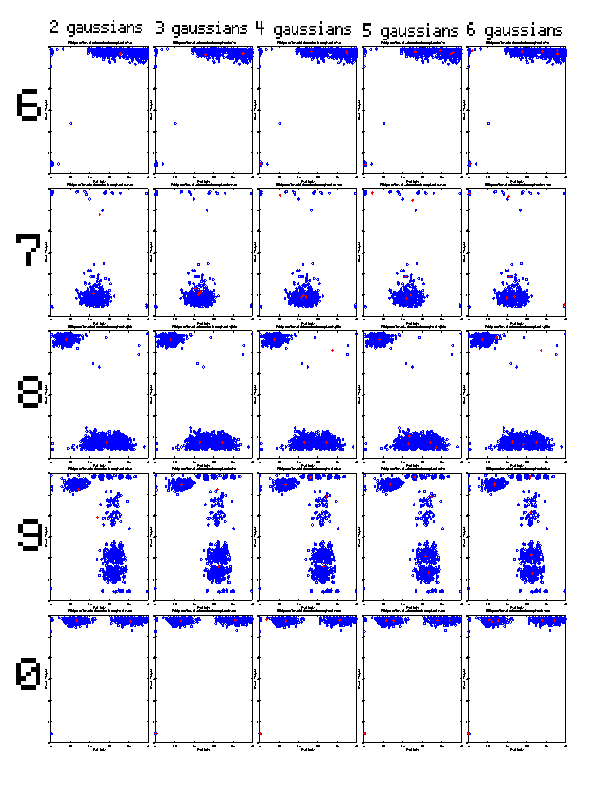Figure 8 plots the centers obtained after 60 iterations (or after convergence) for each of the characters, and each number of centers. To make the graphs easier to read, again, we have hand drawn the centers in purple, and the countours of the clusters of points around each center are sketched (from figure 7). The original untouched graphs can be found in figure 8b. Sometimes the clouds do not show as well as in Figure 7, since we only drew 60 points, instead of 500, to make the centers visible. In red, we drew for each digit the number of centers that we thought would explain the data best (the one we thought had captured the structure of the data the best). In orange are drawn the ones that actually performed best. We were usually off by one center, but sometimes guessed right.

## Evaluating new data

We first tested for each model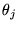, each feature f of a new sample xi independently. The activations from each feature was then combined to form the activation of the character.(12)

since each model evaluates a mixture of gaussians, this is really(13)

for each gaussian g, whereare the parameters of the gth gaussian of the jth character model.

The independence assumption did a very poor job at explaining the data. The character models that covered most territory were explaining away each peak of each test character, and were attributed most of the points.

This is where the power of the hidden markov model comes in. Transition probabilities are calculated on each of the data points. The longer the sequence, the most likely it is to be correctly identified within the framework of a particular model. The probability now becomes(14)

if we know that the first model is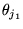, and that the second isand so on. If we don't know the order of the models, we have to sum over all possibilities:(15)

For each character, we evaluate in this fashion every possible configuration for every state within a model. We then choose the maximum activation energy (the highest probability), and we declare the sample as belonging to that character model.

# Results

Results table

## Good performance for long sequences

After the network was trained, we evaluated the data onto the network. We evaluated the entire data first for each character set, with each model. Using the entire data in batch allowed the HMM model to take advantage of the transition probabilities between states that it was trained to recognize. This yielded a very good performance. The log likelihoods are shown in figure 9.

We expect that for each column the maximum number (the least negative) is on the diagonal. This corresponds to saying that a character is best explained by the model that was built on it. This was in fact almost always the case. Only when we got up to 6 centers the model for 5 was explaining one's data best (because the model for 1 went berserk and couldn't explain anything anymore. Classic case of overfitting. 6 centers are probably too many for a character with no peaks).

We should also expect that the diagonal element is also the smallest element on each row. That is, each model is happiest when it meets its own little fellows. This is not always the case though. The 2-state model for 3 is most happy when it sees a 2 then when it sees itself. We guess that this is because there are not enough centers to discriminate among all the features that make a 2 a 2, and not a 3. As the number of states increases, the model for threes always prefers its buddies than the neighbors.

## Individual character results

Different tests were run for individual characters. The performance was quite good for characters with many peaks, but frankly bad for single-peak or double-peak characters. This goes to show that we should have been more careful in choosing a larger number of features to extract from each character. The peaks are not always there, and the orientation, average length, average peak height, peak length, spaces between peaks could have been good features to augment our dimensionality, in case the number of peaks falls down to 1.

The reason why single-peak characters (such as 7) were often misclassified is that performance for a character test depends how well the different gaussians of a model can explain the sample. In fact, when a simple sample arrives to the system, and a complicated model happens to have a center near the peaks of the sample introduced, then the complex model will be able to explain the data. However, the data will not be able to explain the model. That is, some peaks of the model will not be matching any peaks in the data. Hence our criterion for performance could be changed to include also how fit the model is to the sample, or maybe weight a model by how well it does on samples overall, and decrease the score of models that explain any sample well.

# Applications and Future work

## Generating characters from a distribution

The HMM models built can be used to constructing new characters. For example, when creating a personal font set, instead of using the same character every time a'' is entered, the system can sample from a distribution trained on the user's own handwriting.

## Handwriting style recognition

Just like different characters can be recognized across users, the distribution of the characters can be used to recognize users. When data is entered in the computer, the writer can be identified as well, among a set of system users.

## Multi-dimensional data

We could include in the model the widths of the peaks, their average values, the spacing between them, etc, instead of working simply with angles. The orientation of the character could also be included as one of the features to train upon (to separate 8's from).

## Wavelet basis tailored to Characters

Instead of using one of the typical wavelet basis (db4), we can envision using a wavelet basis tailored to the character dataset. We can think that this basis will have primitives for a small curve, a large curve, a loop, and that all those will be easily and compactly representable. Finding the right hi coefficients for an orthogonal wavelet basis for character recognition could greatly benefit the character recognition community.

# Unsupervised Learning

We postulate that a machine learning algorithm could learn to separate the different characters in features space without any class information. It would be interesting to try and construct such uniform clusters from the data. The algorithm could train upon existing data, and construct meaningful clusters. Trained on existing characters, it could then learn to recognize that a new character it has never seen before is a new symbol, being clustered far from all the other symbols. For data to be as easily separable as possible, we would have increase the dimensionality of our feature space. Right now, this dimensionality varies between 8 (when 4 peaks are found) and 2 (single peak) or even 0 (for some cases of 1. uncorrelated data

## Extension to offline data

To find the (dx,dy) increments from the (x,y) points, we used the fact that the order of the points is meaningful. In fact, instead of (x,y) coordinates, we can think of our data as (i,x,y) coordinates where i is the index of the point (enforcing a logical time in the locations of the pen). We don't use the exact times of each point, but simply the order in which they occur. In fact, since some people write slower or faster, and sometimes hesitate at points in the curve, we postulate that our recognition system should be time invariant. Certainly timing is used in signature recognition and has other useful applications, but in our work, it could be more confusing than useful.

An extension of this work could first translate (x,y) pixels obtained from offline data, and recover the ordering for non-crossing characters, by simply joining nearest neighbors. In digits like 1, 3, 5, 7, 0, the pen never crosses itself, so a unique such ordering can be retrieved (up to a reversing that can occur even in online data, and can be dealt with by establishing a normal order on the strokes, cf. Matsakis).

In self-crossing strokes, such as 4, 6, 8, 9, or a looped 2, recovering the order of points can be tricky. Preserving curvature could be used to disambiguate the order of points in a self-crossing 6 or 8, but this heuristic can sometimes fail, since in handwritten character recognition, the curvature can change drastically from one point to the next. In other applications where physical constraints would not allow such high second derivatives, this approach could be used to recover point order.

# Conclusion

We presented a new compact space for doing character recognition. We showed its application to agressive compression and recognition. We evaluated a denoising scheme in this space based on recognition performance. We showed how simple features can be extracted from this space and used in recognition and classification. We used an HMM clustering algorithm to learn Gaussian mixture models for each character, that can classify new characters according to each of the classes. Finally we presented some extensions of the ideas in this work to new applications and new problem domains.

Digit Recognition in Curvature Space

This document was generated using the LaTeX2HTML translator Version 98.1 release (February 19th, 1998)

Copyright © 1993, 1994, 1995, 1996, 1997, Nikos Drakos, Computer Based Learning Unit, University of Leeds.

The command line arguments were:
latex2html chars.tex.

The translation was initiated by Manolis Kamvysselis on 2000-06-11

Manolis Kamvysselis
2000-06-11